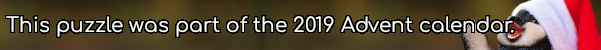mscroggs.co.uk
mscroggs.co.uksubscribe

# Puzzles

## 15 DecemberThere are 5 ways to make 30 by multiplying positive integers (including the trivial way):
• 30
• 2×15
• 3×10
• 5×6
• 2×3×5
Today's number is the number of ways of making 30030 by multiplying.

## 14 DecemberDuring one day, a digital clock shows times from 00:00 to 23:59. How many times during the day do the four digits shown on the clock add up to 14?

## 11 DecemberPut the digits 1 to 9 (using each digit exactly once) in the boxes so that the sums are correct. The sums should be read left to right and top to bottom ignoring the usual order of operations. For example, 4+3×2 is 14, not 10. Today's number is the product of the red digits.
 + ÷ = 2 + ÷ ÷ ÷ ÷ = 3 ÷ - ÷ ÷ ÷ = 1 =2 =1 =1
Tags: grids, numbers

## 9 DecemberArrange the digits 1-9 in a 3×3 square so that: all the digits in the first row are odd; all the digits in the second row are even; all the digits in the third row are multiples of 3; all the digits in the second column are (strictly) greater than 6; all the digits in the third column are non-prime. The number in the first column is today's number.
 all odd all even all multiples of 3 today's number all >6 all non-prime
Tags: numbers, grids

## 8 DecemberCarol uses the digits from 0 to 9 (inclusive) exactly once each to write five 2-digit even numbers, then finds their sum. What is the largest number she could have obtained?
Tags: numbers

## 6 DecemberNoel's grandchildren were in born in November in consecutive years. Each year for Christmas, Noel gives each of his grandchildren their age in pounds.
Last year, Noel gave his grandchildren a total of £208. How much will he give them in total this year?

## 4 DecemberThere are 5 ways to tile a 3×2 rectangle with 2×2 squares and 2×1 dominos.
Today's number is the number of ways to tile a 9×2 rectangle with 2×2 squares and 2×1 dominos.

## 3 DecemberPut the digits 1 to 9 (using each digit exactly once) in the boxes so that the sums are correct. The sums should be read left to right and top to bottom ignoring the usual order of operations. For example, 4+3×2 is 14, not 10. Today's number is the largest number you can make with the digits in the red boxes.
 + + = 21 + × × + + = 10 + ÷ × + + = 14 =21 =10 =14
Tags: numbers, grids

## Archive

Show me a random puzzle
▼ show ▼